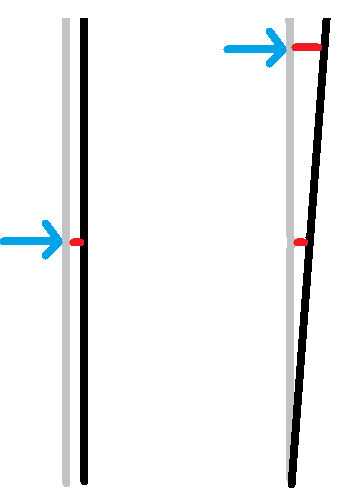• B
• kuruman
B for Case 1; C for Case 2]In summary, the wheel will be moved to the left in Case 1, and to the right in Case 2.
jbriggs444 said:

Energy goes as force times displacement. Momentum goes as force times time. The two need not be proportional. With an off-center hit there is less resistance at the point of impact, so more velocity at the point of impact, so more displacement at the point of impact, so more energy transferred for a given impulse.
So I can take my constant impulse and potentially create a more kinetic energy from said impulse depending on how I apply it? I'm guessing the same impulse will create more energy if the distance traveled is larger, so that would take more effort on my part, the person applying the force. The puzzle is starting to come together. Thank you.

•jbriggs444
Chenkel said:
So I can take my constant impulse and potentially create a more kinetic energy from said impulse depending on how I apply it? I'm guessing the same impulse will create more energy if the distance traveled is larger, so that would take more effort on my part, the person applying the force. The puzzle is starting to come together. Thank you.

Same force, but applied to CM vs (almost at the) tip of the rod. The time of impact is also the same, hence same impulse. When force is applied to the tip of the rod, the displacement is longer, thus more work is done.•Chenkel, erobz and Orodruin
Chenkel said:
So I can take my constant impulse and potentially create a more kinetic energy from said impulse depending on how I apply it? I'm guessing the same impulse will create more energy if the distance traveled is larger, so that would take more effort on my part, the person applying the force. The puzzle is starting to come together. Thank you.
Simply put: Impulse is not energy. It is therefore not a given that a particular impulse should correspond to a particular energy.

The details have been discussed above.

•Chenkel and erobz